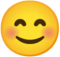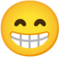## Hundred NumbersPuzzle

Visible to anyone in the world
Edited by Richard Walker, Sunday, 28 Aug 2022, 10:46
Suppose someone is going to read out to you the numbers 1-100 in random order, but they will miss one out. When they have finished you will be asked which number was missed.

You have no means of recording the numbers as they are read, and although you are quite good at mental arithmetic you only have an average memory and remembering the entire list of numbers as they were read out is beyond you.

What is the best way to identify the missing number?
Share post

### New comment

Sum the numbers as they are read out, and subtract the total from 5050 to give the missing number?

Not that I could do it in my head.  I'm totally useless at mental arithmetic and poor at number memory too.

Jan

### New comment

Spot-on!

What if two numbers were missing### New comment

Oh dear... that's probably stretching my dyscalculic brain a bit too far.

But I may come back to that one later.

Jan.

### New comment

Hmmm

I suspect it can't be done by my method so far,

as there is more than one way to split the shortfall into 2 smaller numbers.

Except for a shortfall of 3, when the missing numbers would be 1 and 2.

Jan

### New comment

Well done Jan. 🙂

Hi Richard,

If the person hearing the numbers being read out remembers them then s/he simply states the two missing numbers. If, however, that person is given the sum of the 98 numbers then that number is unique to the combination of numbers which make it up. It probably wouldn't take too long to find that combination and that would reveal the two missing numbers.

Another way to find the two missing numbers is to subtract the given sum from 5050 and the remainder will be made up of two unique numbers - the missing ones.

Seems logical to me but I'm open to correction.

### New comment

If the shortfall was say 20 then the missing pair might be 19 and 1, 18 and 2, etc. So finding which is the right pair needs a bit more work.

Remembering the 98 numbers and then mentally checking the list to see which pair are absent would be pretty tough. So we need a better way.

### New comment

I don't think we can find the 2 missing numbers (except pairs 1,2 and 1,3) unless we are given more information.  I'm pondering what information to ask for.

Otherwise, with no additional information, a different method is needed, possibly involving multiplication and/or division to find factors of something.

Inspiration may strike during the day 🤔.

Jan

### New comment

Hi Richard,

Yes, I can see the obvious (now that you have pointed it out), which was obscured to me by my thinking that each of the 98 sets of numbers chosen would yield a unique sum. Perhaps they do? I am stimulated to ask myself 'How many combinations of 98 numbers out of 100 can be summed with a unique answer? Is the answer 98? Or more? I am 'drowning myself' - happily so, in what to me, seems a miasma of rhetorical questions. Nevertheless, I am no nearer to a solution!!

So, a number uniquely tied to another number in such a way as to be impossible to replicate in another coupling?

Very interesting question, the answer to which I look forward to seeing!!

### New comment

How many combinations of 98 numbers out of 100 ...?

Should have added 'from 1 to 100'.

### New comment

>> How many combinations of 98 numbers out of 100 can be summed with a unique answer?

It's confusing to think about, but I believe the only unique sums are

3, 4, 5, ..., 100

2, 4, 5, ..., 100

1, 2, 3, ..., 98, 100

1, 2, 3, ..., 99, 100

Rich### New comment

In fact as long as 1 ≤ n - 1 and n + 2  ≤ 100, so all the numbers involved are in the range 1...100,  missing out the pair n and n + 1 will give the same result as missing out n - 1 and n + 2; both will give a total of 5050  - (2n + 1).

Rich### New comment

Here's how you could identify two missing numbers, although the mental effort would be considerable!

Keep a running total of numbers and of their squares. At the end take the total from 5050 and the total of squares from 338350. Now you know the sum of the missing numbers and the sum of their squares. Call these M and N and suppose the missing numbers are x and y, so

x + y = M

x2 + y2 = N

Some algebra tell us that (M2 - N) = xy, so by calculating this quantity we find the product of the missing numbers. We can factor this product and list the possibilities for x and y. There may be several and if so we can identify the correct pair by looking for the one which gives the right sum.

For example, if M = 31 and N = 625 we have (M2 - N)/2 = 168 and 168 = 7 x 24, so in this case the missing numbers can only be 7 and 24, and we can check that 7 + 24 = 31.

### New comment

Thanks Richard,

I hadn't given it any more thought since my last comment, but very interesting to see that it is possible without more information.

Definitely beyond my mental arithmetic capability. I have difficulty with very simple addition and subtraction in my head (and diagnosed with a learning disability).  But that's why I programmed computers for a livingI can usually understand the methods, but just can't do it myself.

Jan

### New comment

Thanks for that Richard. Very interesting to see how such a difficult problem could be solved.

The great 20th century mathematician Paul Erdős is said to have had that kind of ability. In his biography The Man Who Loved Only Numbers there is an account of him going to a telephone directory and memorising many numbers at once.

Joseph.